Like   Tweet   Pin/* styles */ 20 October 2020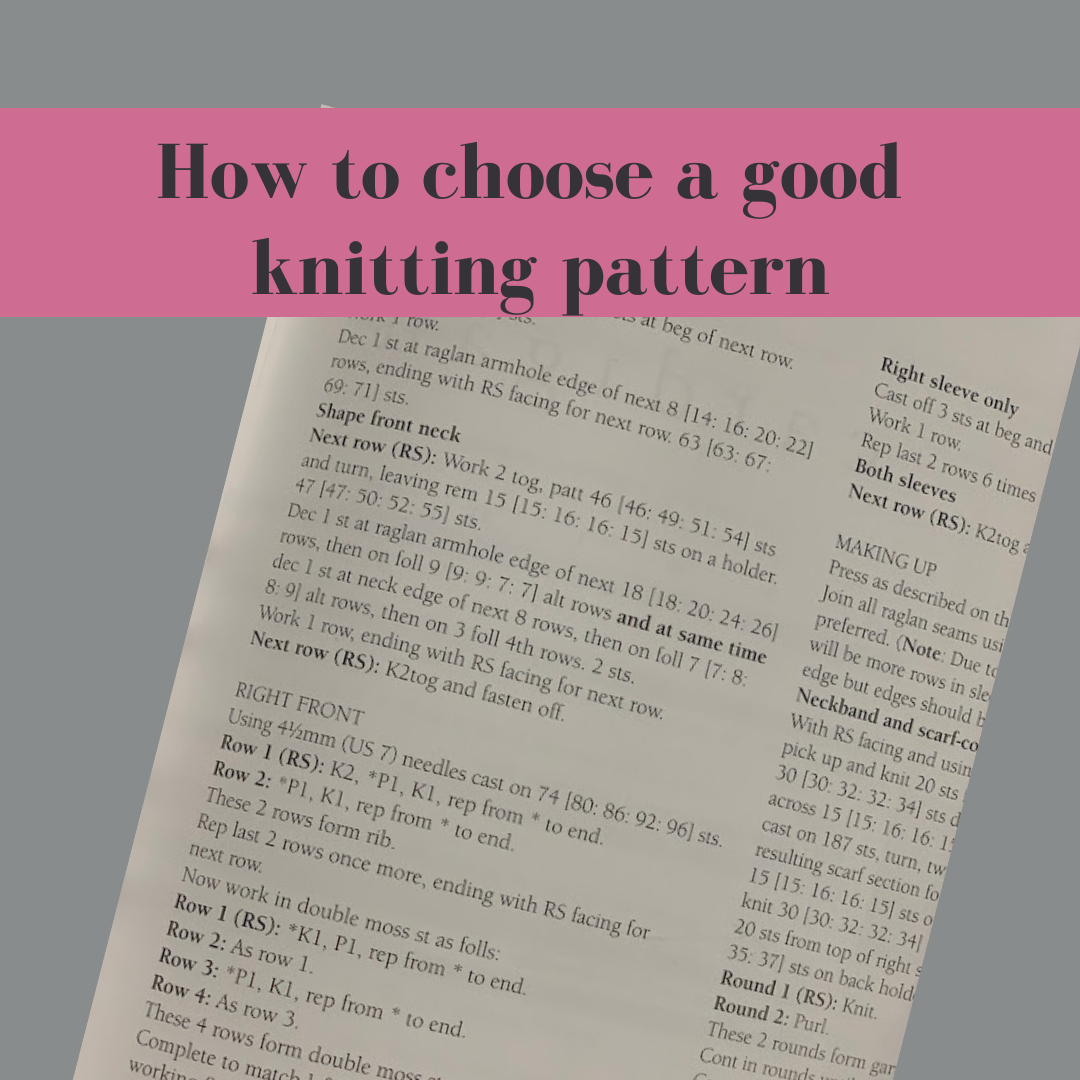Warren Buffet is famously quoted as saying, If you don’t know jewels, know your jeweler. My mother said similar things regarding all sorts of professionals. From butchers to car mechanics, if you are not an expert, find someone who is, and put your trust in them. The true professionals have a great deal of pride in their work and take genuine pleasure in helping you—in educating you, really, in that beautiful intersection of your interest and their expertise. The charlatans who knowingly dupe you are especially vile, but the amateurs who accidentally mess you up, can be just as bad.

I see this on Ravelry all the time. As I often say, the wonderful things about Ravelry is that anyone can be a designer, but the really bad thing about Ravelry is that anyone continue reading about choosing a good knitting pattern

## Casapinka Glamping Blanket kit!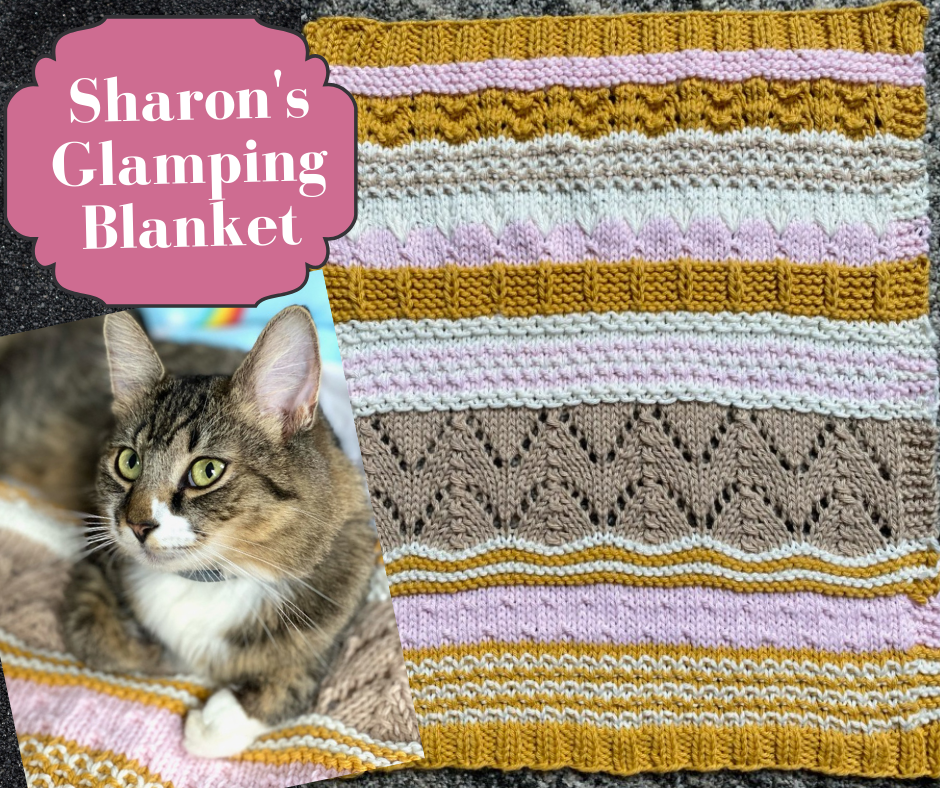Sharon, Casapinka's fierce feline Head of Security designed this cozy blanket in soft, washable Berroco Vintage for fun Glamping trips and also for happy time at home beside the fire. There are four colors and four sizes available

cat 18"x22"
Baby, small dog, portly cat 24"x36"
Dog/lap blanket 35"x44"
Sofa blanket for humans 46"x58"

For more details and to order, see Casapinka Glamping Kit Afghan

 table div table+table+table+table+table+table+table+table+table div table{width:100%;padding:0}table div table+table+table+table+table+table+table+table+table div table table{padding:0;float:left!important;width:26.037%!important}table div table+table+table+table+table+table+table+table+table div table table+table+table+table td,table div table+table+table+table+table+table+table+table+table div table td{padding-left:0;padding-right:0}table div table+table+table+table+table+table+table+table+table div table table+table{float:left!important;width:26.037%!important}table div table+table+table+table+table+table+table+table+table div table table td,table div table+table+table+table+table+table+table+table+table div table table+table td,table div table+table+table+table+table+table+table+table+table div table table+table+table td{padding-left:0;padding-right:20px}table div table+table+table+table+table+table+table+table+table div table table+table+table{float:left!important;width:25.849%!important}table div table+table+table+table+table+table+table+table+table div table table+table+table+table{float:left!important;width:22.076999999999998%!important}/* styles */
 table div table+table+table+table+table+table+table+table+table+table div table{width:100%;padding:0}table div table+table+table+table+table+table+table+table+table+table div table img{width:96.23%;padding:0;float:none}table div table+table+table+table+table+table+table+table+table+table div table td{width:100%;padding:0 1.88% 18px}/* styles */## Nancy Shawl - Boo Knits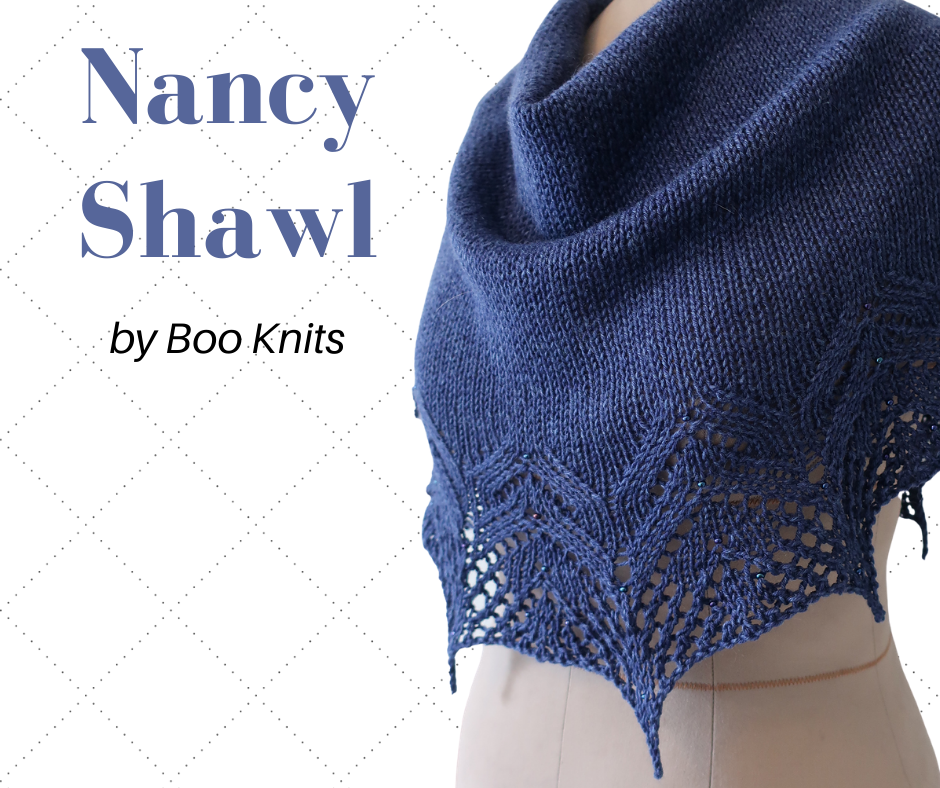Meet Nancy, by Boo Knits. I love this shawl pattern, and I've been looking for a perfect partner for beautiful new Katia Infinity. I think Nancy is the one. A beautifully written pattern, both charted and written out, it is easy to follow, and deceptively easy to knit. I think it will be especially amazing in Inifinty Shawl's gradient colors.

 table div table+table+table+table+table+table+table+table+table+table+table+table+table div table{width:100%;padding:0}table div table+table+table+table+table+table+table+table+table+table+table+table+table div table table{padding:0;float:left!important;width:26.037%!important}table div table+table+table+table+table+table+table+table+table+table+table+table+table div table table+table+table+table td,table div table+table+table+table+table+table+table+table+table+table+table+table+table div table td{padding-left:0;padding-right:0}table div table+table+table+table+table+table+table+table+table+table+table+table+table div table table+table{float:left!important;width:26.037%!important}table div table+table+table+table+table+table+table+table+table+table+table+table+table div table table td,table div table+table+table+table+table+table+table+table+table+table+table+table+table div table table+table td,table div table+table+table+table+table+table+table+table+table+table+table+table+table div table table+table+table td{padding-left:0;padding-right:20px}table div table+table+table+table+table+table+table+table+table+table+table+table+table div table table+table+table{float:left!important;width:25.849%!important}table div table+table+table+table+table+table+table+table+table+table+table+table+table div table table+table+table+table{float:left!important;width:22.076999999999998%!important}/* styles */
 table div table+table+table+table+table+table+table+table+table+table+table+table+table+table div table{width:100%;padding:0}table div table+table+table+table+table+table+table+table+table+table+table+table+table+table div table table{padding:0;float:left!important;width:26.037%!important}table div table+table+table+table+table+table+table+table+table+table+table+table+table+table div table table+table+table+table td,table div table+table+table+table+table+table+table+table+table+table+table+table+table+table div table td{padding-left:0;padding-right:0}table div table+table+table+table+table+table+table+table+table+table+table+table+table+table div table table+table{float:left!important;width:26.037%!important}table div table+table+table+table+table+table+table+table+table+table+table+table+table+table div table table td,table div table+table+table+table+table+table+table+table+table+table+table+table+table+table div table table+table td,table div table+table+table+table+table+table+table+table+table+table+table+table+table+table div table table+table+table td{padding-left:0;padding-right:20px}table div table+table+table+table+table+table+table+table+table+table+table+table+table+table div table table+table+table{float:left!important;width:25.849%!important}table div table+table+table+table+table+table+table+table+table+table+table+table+table+table div table table+table+table+table{float:left!important;width:22.076999999999998%!important}/* styles */
 table div table+table+table+table+table+table+table+table+table+table+table+table+table+table+table div table{width:100%;padding:0}table div table+table+table+table+table+table+table+table+table+table+table+table+table+table+table div table img{width:96.23%;padding:0;float:none}table div table+table+table+table+table+table+table+table+table+table+table+table+table+table+table div table td{width:100%;padding:0 1.88% 18px}/* styles */## Crazy for Ewe Live is now a Video Podcast!

Early this year I talked about starting a podcast. I was all in and ready to go. Then everything went all 2020 on me. I started doing the Crazy for Ewe Live events, to say hi and stay in touch. That turned into a regularly scheduled thing with guests and a format, and well, a video podcast! So look for it on the Crazy for Ewe Yarns Facebook Page and the Crazy for Ewe YouTube Channel Share it on Facebook with your friends, subscribe to the YouTube Channel, and click the little bell icon so you can get a reminder when each episode is about to go live.

I'm so happy and proud to have been able to bring you great guests and patterns on the podcast, and I hope you enjoy getting a little inside scoop on the industry and more. Thanks for watching, and for being part of the community.

# This Week's Episode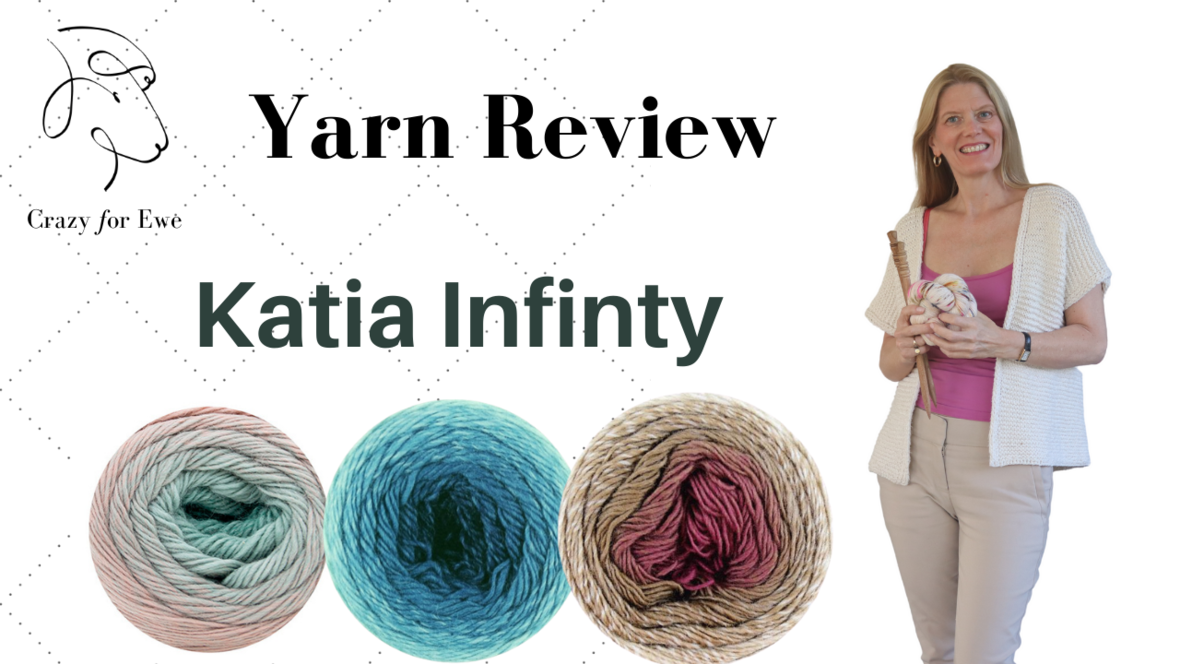table div table+table+table+table+table+table+table+table+table+table+table+table+table+table+table+table+table+table+table div table{width:100%;padding:0}table div table+table+table+table+table+table+table+table+table+table+table+table+table+table+table+table+table+table+table div table img{width:96.23%;padding:0;float:none}table div table+table+table+table+table+table+table+table+table+table+table+table+table+table+table+table+table+table+table div table td{width:100%;padding:0 1.88% 18px}/* styles */## Interested in the sweater class?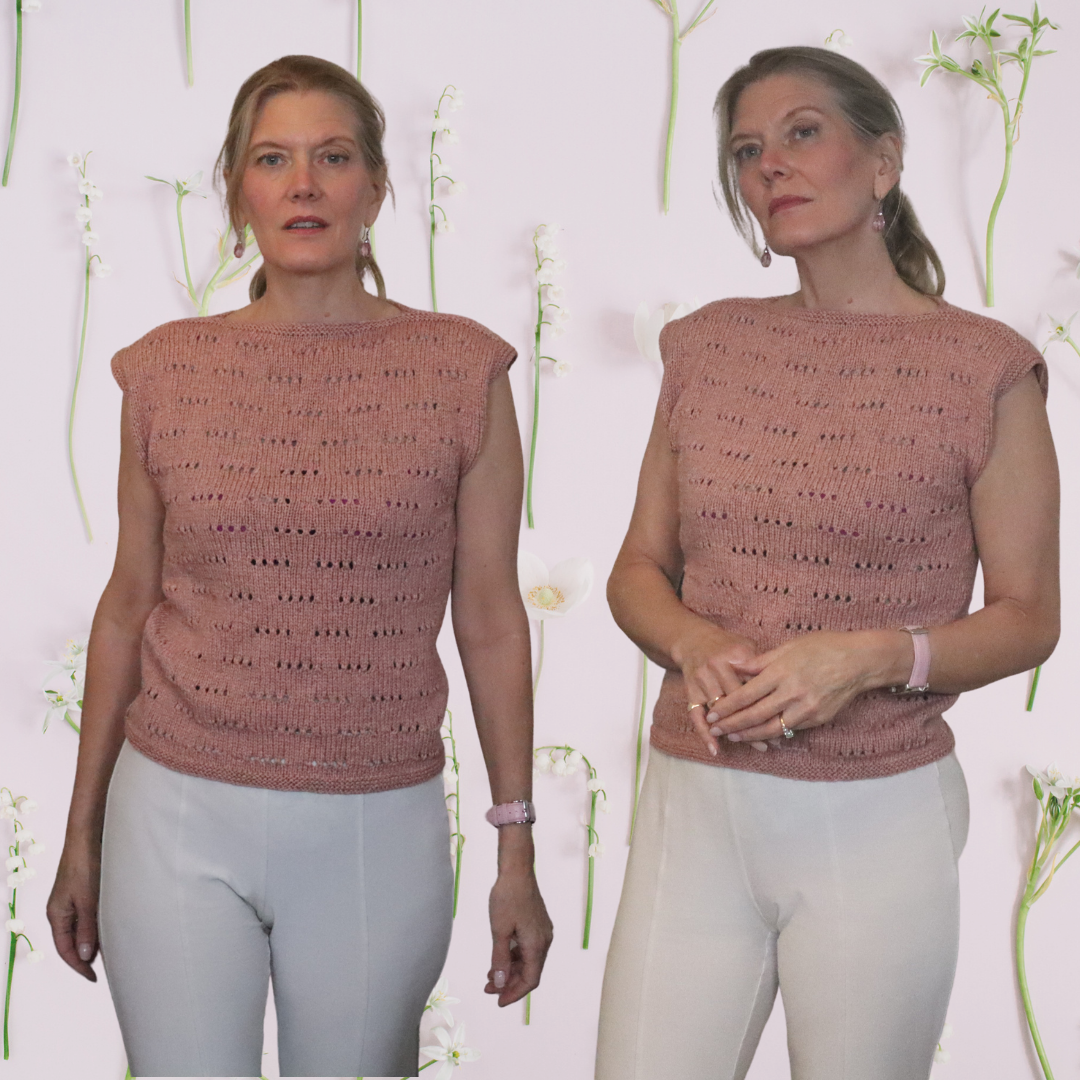# Last chance to purchase at this price

A comprehensive course that will have you wearing a beautiful top you made yourself. I'll be right there with you, every step of the way-- from working your gauge swatch to weaving in your ends. Detailed instruction, supportive guidance, and patient support will ensure you learn everything you need to make this cute top.

 table div table+table+table+table+table+table+table+table+table+table+table+table+table+table+table+table+table+table+table+table+table+table div table{width:100%;padding:0}table div table+table+table+table+table+table+table+table+table+table+table+table+table+table+table+table+table+table+table+table+table+table div table img{width:96.23%;padding:0;float:none}table div table+table+table+table+table+table+table+table+table+table+table+table+table+table+table+table+table+table+table+table+table+table div table td{width:100%;padding:0 1.88% 18px}/* styles */